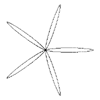j3d.org Code

org.j3d.geom Class SuperShapeGenerator

java.lang.Objectorg.j3d.geom.GeometryGeneratororg.j3d.geom.SuperShapeGenerator

public class SuperShapeGenerator
extends GeometryGenerator

Generalised shape generator that is capable of generating almost any 2D shape using a standard algorithm.

This class generates 3D coordinates for a single flat object in the X,Y plane with a Z value of zero. Because this is only generating 2D shapes in reality, it only supports the LINE_ geometry types. All Triangle and quad forms are not supported.

The basic equation for a supershape comes from the standard form that you are familiar with for any ellipsoid.

(x / a)^2 + (y / b)^2 = r^2

Turning this into polar coordinates, the equation becomesBelow are some example shapes from the various coordinates.
 m = 0. This results in circles, namely r = 1n1 = n2 = n3 = 1 Increasing m adds rotational symmetry to the shape. This is generally the case for other values of the n parameters. The curves are repeated in sections of the circle of angle 2 * pi/m, this is apparent in most of the following examples for integer values of m. m = 1 m = 2 m = 3 m = 4 m = 5 m = 6If n1 is slightly larger than n2 and n3 then bloated forms result. The examples on the right have n1 = 40 and n2 = n3 = 10. m = 1 m = 2 m = 3 m = 4 m = 5 m = 6Polygonal shapes are achieved with very large values of n1 and large but equal values for n2 and n3. m = 3, n1 = 1000, n2 = 1980, n3 = 1980 m = 4, n1 = 1000, n2 = 1000, n3 = 1000 m = 5, n1 = 1000, n2 = 620, n3 = 620 m = 6, n1 = 1000, n2 = 390, n3 = 390Asymmetric forms can be created by using different values for the n's. The following example have n1 = 60, n2 = 55 and n3 = 30. m = 3 m = 4 m = 5 m = 6For non integral values of m the form is still closed for rational values. The following are example with n1 = n2 = n3 = 0.3. The max angle needs to extend from 0 to 12 pi. m = 1/6 m = 7/6 m = 13/6 m = 19/6Smooth starfish shapes result from smaller values of n1 than the n2 and n3. The following examples have m=3 and n2 = n3 = 1.7. n1=0.50 n1=0.20 n1=0.10 n1=0.02The original idea as well as all the pictures are stolen from Paul Bourke's SuperShape page: http://astronomy.swin.edu.au/~pbourke/curves/supershape/

Version:
\$Revision: 1.2 \$
Author:
Justin Couch

Constructor Summary
SuperShapeGenerator()
Construct a new generator with default settings of 10 divisions over a single side between two control points.
SuperShapeGenerator(int facets)
Construct a new generator with the specified number of tessellations over the side of the curve.

Method Summary
void generate(GeometryData data)
Generate a new set of geometry items patchd on the passed data.
int getVertexCount(GeometryData data)
Get the number of vertices that this generator will create for the curve.
void setCoefficients(double m, double n1, double n2, double n3, double a, double b)
Set the coefficient values to generate a new shape.
void setFacetCount(int facets)
Change the number of facets used to create this cone.
void setMaxAngle(double angle)
Set the maximum angle to use for phi.

Methods inherited from class org.j3d.geom.GeometryGenerator

Methods inherited from class java.lang.Object
clone, equals, finalize, getClass, hashCode, notify, notifyAll, toString, wait, wait, wait

Constructor Detail

SuperShapeGenerator

public SuperShapeGenerator()
Construct a new generator with default settings of 10 divisions over a single side between two control points.

SuperShapeGenerator

public SuperShapeGenerator(int facets)
Construct a new generator with the specified number of tessellations over the side of the curve. The default coefficients will generate a circle. The maximum angle defaults to 2 * pi.

Parameters:
facets - The number of facets on a segment of the curve
Throws:
java.lang.IllegalArgumentException - The number of divisions is less than 3
Method Detail

setFacetCount

public void setFacetCount(int facets)
Change the number of facets used to create this cone. This will cause the geometry to be regenerated next time they are asked for. The minimum number of facets is 3.

Parameters:
facets - The number of facets on the side of the cone
Throws:
java.lang.IllegalArgumentException - The number of facets is less than 3

setCoefficients

public void setCoefficients(double m,
double n1,
double n2,
double n3,
double a,
double b)
Set the coefficient values to generate a new shape. The values a and b must not be zero and will generate an error if they are.

setMaxAngle

public void setMaxAngle(double angle)
Set the maximum angle to use for phi. Set separately because for the m most part, this will be a value of 2 * pi and never change. No error checking is performed.

Parameters:
angle - The maximum angle to use in radians

getVertexCount

public int getVertexCount(GeometryData data)
throws UnsupportedTypeException
Get the number of vertices that this generator will create for the curve. This is just the number of facets + 1.

Specified by:
getVertexCount in class GeometryGenerator
Parameters:
data - The data to base the calculations on
Returns:
The vertex count for the object
Throws:
UnsupportedTypeException - The generator cannot handle the type of geometry you have requested

generate

public void generate(GeometryData data)
throws UnsupportedTypeException,
InvalidArraySizeException
Generate a new set of geometry items patchd on the passed data. If the data does not contain the right minimum array lengths an exception will be generated. If the array reference is null, this will create arrays of the correct length and assign them to the return value.

Specified by:
generate in class GeometryGenerator
Parameters:
data - The data to patch the calculations on
Throws:
InvalidArraySizeException - The array is not big enough to contain the requested geometry
UnsupportedTypeException - The generator cannot handle the type of geometry you have requested

j3d.org Code

Latest Info from http://code.j3d.org/